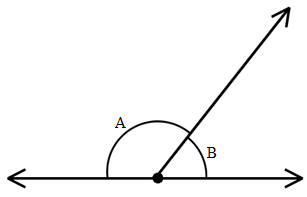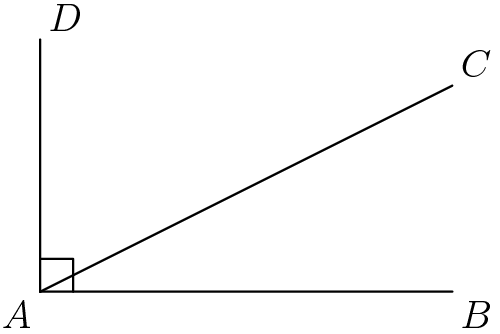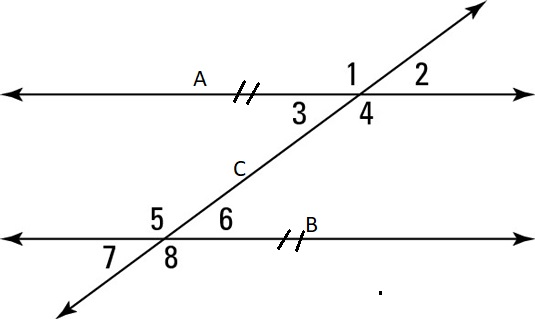# Angles

Angles represent the space between two lines (measured in degrees). Let's take a look at different types of angles.

Different types of angles
Type Description
Acute Angle it is less than 90°
Right Angle it is exactly 90°
Obtuse Angle it is greater than 90° but less than 180°
Straight Angle it is exactly 180°
Reflex Angle it is greater than 180° but less than 360°
Full Rotation it is 360° exactly

## Opposite Angles

Opposite angles are across the transversal. Opposite angles are equal.## Corresponding Angles

Corresponding angles are in the same position relative to lines intersected by a transversal. Corresponding angles are equal when the two lines intersected by the transversal are parallel.## Alternate Angles

Alternate angles are in opposite positions relative to a transversal intersecting two lines. Alternate angles are equal when the two lines intersected by the transversal are parallel.## Co-interior Angles

Co-interior angles are on the same side of an transversal line when it intersect two other lines. Co-interior angles add to $$180^\circ$$.## Supplementary Angles

Two angles are supplementary when they add up to 180 degrees (they make a straight line).

$$\angle A$$ and $$\angle B$$ below form a straight line so they must add to 180 degrees.## Complementary Angles

Two angles are complementary when they add up to 90 degrees (a right angle).

$$\angle{DAC}$$ and $$\angle{CAB}$$ form a right angle and would add up to 90 degrees.Refer to the figure below. Guess which angles correspond with each type then press the button to show the answer.What angle is supplementary to $$40^\circ$$?

An angle is double its complementary angle. What is the value of the larger angle?

A straight line is divided into 10 equal angles. How big is each angle?